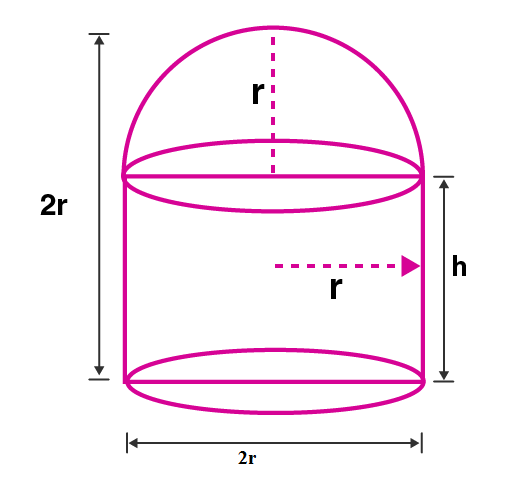Newbie

# A circular hall (big room) has a hemispherical roof. The greatest height is equal to the inner diameter, find the area of the floor, given that the capacity of the hall is 48510 m cube.

• 0

M.L Aggarwal book Important Question of class 10 chapter Based on Mensuration for ICSE BOARD.
A circular hall has a hemispherical roof. The greatest height is equal to the inner diameter, find the area of the floor, the capacity of the hall is given.
This is the Question Number 16, Exercise 17.4 of M.L Aggarwal.

Share

1.Let the radius of the hemisphere be r.

Inner diameter = 2r

Given greatest height equal to inner diameter.

So total height of the hall = 2r

Height of the hemispherical part = r

Height of cylindrical area, h = 2r-r = r

Capacity of the hall = Volume of cylindrical area + volume of hemispherical area

= r2h + (2/3)r3

= r3+ (2/3)r3 [∵h = r]

= r(1+2/3)

= r(3+2)/3

= (5/3)r3

Given capacity of hall = 48510 m3

(5/3)r3= 48510

(5/3)×(22/7)r3= 48510

r3 = 48510×3×7/(22×5)

r3 = 9261

Taking cube root on both sides,

r = 21

Area of the floor = r2

= (22/7)×212

= (22×21×3

= 1386 m2

Hence the Area of the floor is 1386 m2 .

• 1